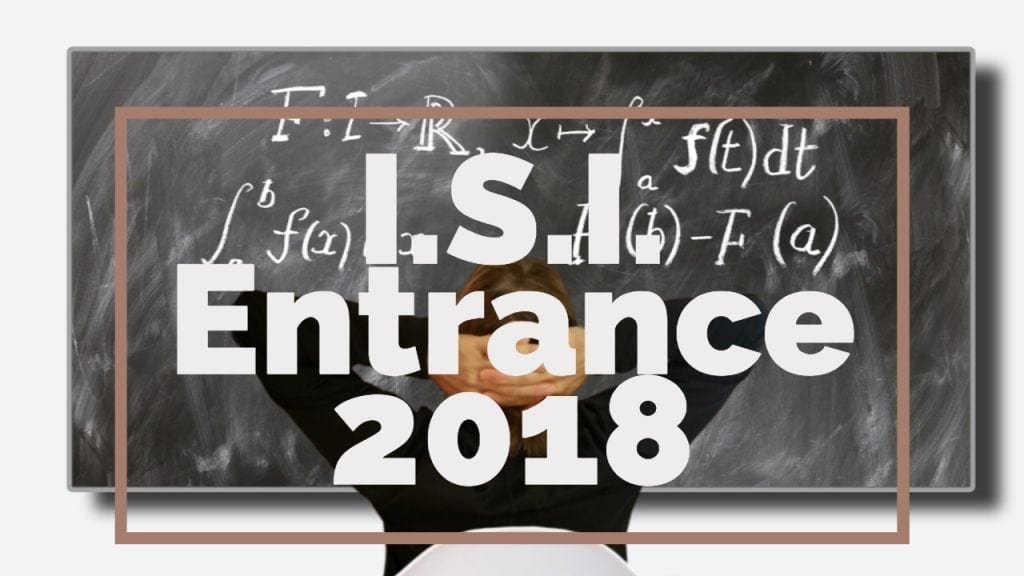How Cheenta works to ensure student success?
Explore the Back-Story

# ISI Entrance Paper 2018 - B.Stat, B.Math SubjectiveHere, you will find all the questions of ISI Entrance Paper 2018 from Indian Statistical Institute's B.Stat Entrance. You will also get the solutions soon of all the previous year problems.

Problem 1:

Find all pairs $(x,y)$ with $x,y$ real, satisfying the equations:

$\sin(\frac{x+y}{2})=0,\vert x\vert+\vert y\vert=1$

Problem 2:

Suppose that $PQ$ and $RS$ are two chords of a circle intersecting at a point $O$. It is given that $PO=3 \mathrm{cm}$ and $SO=4 \mathrm{cm}$. Moreover, the area of the triangle $POR$ is $7 \mathrm{cm}^2$. Find the area of the triangle $QOS$.

Problem 3:

Let $f:\mathbb{R} \to \mathbb{R}$ be a continuous function such that for all $x \in \mathbb{R}$ and for all $t \geq 0$, $f(x)=f(e^{t}x)$. Show that $f$ is a constant function.

Problem 4:

Let $f:(0,\infty)\to \mathbb{R}$ be a continuous function such that for all $x \in(0,\infty)$, $f(2x)=f(x)$. Show that the function $g$ defined by the equation $g(x)=\int_{x}^{2x} f(t)\frac{dt}{t}$ for $x>0$ is a constant function.

Problem 5:

Let $f:\mathbb{R}\to \mathbb{R}$ be a differentiable function such that its derivative $f'$ is a continuous function. Moreover, assume that for all $x \in\mathbb{R}$, $0 \leq \vert f'(x)\vert\leq \frac{1}{2}$. Define a sequence of real numbers $\{a_n\}_{n\in\mathbb{N}}$ by : $a_1=1$ and $a_{n+1}=f(a_n)$ for all $n\in\mathbb{N}$. Prove that there exists a positive real number $M$ such that for all $n\in\mathbb{N}$,

|an|M

Problem 6:

Let, $a\geq b\geq c >0$ be real numbers such that for all natural number $n$, there exist triangles of side lengths $a^{n} , b^{n} ,c^{n}$. Prove that the triangles are isosceles.

Problem 7:

Let $a, b, c$ are natural numbers such that $a^{2}+b^{2}=c^{2}$ and $c-b=1$

Prove that,

(i) $a$ is odd,

(ii) $b$ is divisible by $4$,

(iii) $a^{b}+b^{a}$ is divisible by $c$.

Problem 8:

Let $n\geq 3$. Let $A=((a_{ij}))_{1\leq i,j\leq n}$ be an $n\times n$ matrix such that $a_{ij}\in\{-1,1\}$ for all $1\leq i,j\leq n$. Suppose that $a_{k1}=1$ for all $1\leq k\leq n$ and $\sum_{k=1}^n a_{ki}a_{kj}=0$ for all $i\neq j$. Show that $n$ is a multiple of $4$.

Here, you will find all the questions of ISI Entrance Paper 2018 from Indian Statistical Institute's B.Stat Entrance. You will also get the solutions soon of all the previous year problems.

Problem 1:

Find all pairs $(x,y)$ with $x,y$ real, satisfying the equations:

$\sin(\frac{x+y}{2})=0,\vert x\vert+\vert y\vert=1$

Problem 2:

Suppose that $PQ$ and $RS$ are two chords of a circle intersecting at a point $O$. It is given that $PO=3 \mathrm{cm}$ and $SO=4 \mathrm{cm}$. Moreover, the area of the triangle $POR$ is $7 \mathrm{cm}^2$. Find the area of the triangle $QOS$.

Problem 3:

Let $f:\mathbb{R} \to \mathbb{R}$ be a continuous function such that for all $x \in \mathbb{R}$ and for all $t \geq 0$, $f(x)=f(e^{t}x)$. Show that $f$ is a constant function.

Problem 4:

Let $f:(0,\infty)\to \mathbb{R}$ be a continuous function such that for all $x \in(0,\infty)$, $f(2x)=f(x)$. Show that the function $g$ defined by the equation $g(x)=\int_{x}^{2x} f(t)\frac{dt}{t}$ for $x>0$ is a constant function.

Problem 5:

Let $f:\mathbb{R}\to \mathbb{R}$ be a differentiable function such that its derivative $f'$ is a continuous function. Moreover, assume that for all $x \in\mathbb{R}$, $0 \leq \vert f'(x)\vert\leq \frac{1}{2}$. Define a sequence of real numbers $\{a_n\}_{n\in\mathbb{N}}$ by : $a_1=1$ and $a_{n+1}=f(a_n)$ for all $n\in\mathbb{N}$. Prove that there exists a positive real number $M$ such that for all $n\in\mathbb{N}$,

|an|M

Problem 6:

Let, $a\geq b\geq c >0$ be real numbers such that for all natural number $n$, there exist triangles of side lengths $a^{n} , b^{n} ,c^{n}$. Prove that the triangles are isosceles.

Problem 7:

Let $a, b, c$ are natural numbers such that $a^{2}+b^{2}=c^{2}$ and $c-b=1$

Prove that,

(i) $a$ is odd,

(ii) $b$ is divisible by $4$,

(iii) $a^{b}+b^{a}$ is divisible by $c$.

Problem 8:

Let $n\geq 3$. Let $A=((a_{ij}))_{1\leq i,j\leq n}$ be an $n\times n$ matrix such that $a_{ij}\in\{-1,1\}$ for all $1\leq i,j\leq n$. Suppose that $a_{k1}=1$ for all $1\leq k\leq n$ and $\sum_{k=1}^n a_{ki}a_{kj}=0$ for all $i\neq j$. Show that $n$ is a multiple of $4$.

This site uses Akismet to reduce spam. Learn how your comment data is processed.

### 11 comments on “ISI Entrance Paper 2018 - B.Stat, B.Math Subjective”

1.Yash says:

I have the objective qus paper and I wish to see ur solution on those qus..
Can I have ur fb account or whatsApp no. I can send u pics of qus paper

2.Ravesh kumar says:

Plz regularly update me.

1.Ashani Dasgupta says:

Sure

3.MOUSAM BISWAS says:

Please someone provide me with the objective question paper of this entrance test without solution

4.MOUSAM BISWAS says:

plz provide the obj. question paper

5.Ashis Dey says:

Sir in question 4 isi subjective paper it is a constant function

6.Ashis Dey says:

Sir isi question 4 subjective is a constant function

7.Rohan Bhattacharya says:

Please give me the solution of problem no 5

8.SN says:

Regarding the hint for Problem 8, det(n I) = n^n, not n. Is this an intentional mistake?

1.Ashani Dasgupta says:

Yes that is a typo. We will post a complete discussion soon.

### Knowledge Partner